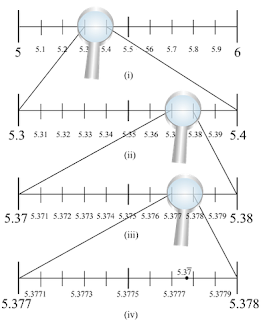#### Chapter 1 Number System R.D. Sharma Solutions for Class 9th Math Exercise 1.6

Exercise 1.6

1. Visualise 2.665 on the number line, using successive magnification.

SolutionWe know that 2.665 lies between 2 and 3. So, we divide the number line into 10 equal parts
and mark each point of division. The first mark on the right of 2 will  be 2.1 followed by 2.2 and so on. The point left of 3 will be 2.9. Now, the magnified view of this will show that 2.665 lies between 2.6 and2.7. So, our focus will be now 2.6 and 2.7. We divide this again into 10 equal parts. The first part will be2.61 followed by 2.62 and so on. We now magnify this again and find that 2.665 lies between 2.66 and 2.67. So, we magnify this portion and divide it again into 10 equal parts. The first part will represent 2.661, next will be 2.662 and so on.So, 2.665 will be 5th mark in this subdivision as shown in the figure.

2. Visualise the representation of 5.37 on the number line upto 5 decimal place, that is upto 5.37777

SolutionFirst, we see that 5.37 is located between 5 and 6. In the next step, we locate 5.37 between 5.3 and 5.4. To get a more accurate visualization of the representation, we divide this portion of the number line into 10 equal parts and use a magnifying glass to visualize that 5.37 lies between 5.37 and 5.38. To visualize 5.37 more accurately, we again divide the portion between 5.37 and 5.38 into ten equal parts and use a magnifying glass to visualize that 5.37 lies between 5.377 and 5.378. Now to visualize 5.37 still more accurately, we divide the portion between 5.377 and 5.378 into 10 equal parts, and visualize the representation of 5.37 as in Fig. 1.3 (iv). We notice that 5.37 is located closer to 5.3778 than to 5.3777.